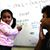S. Prem Kumar
3

To add to the answer already provided, you first need to define what you mean by 'energy' -- as has already been said, as it stands it is just a word and you need a definition.

Depending on the level of refinement you want to choose, energy can be defined in various ways, all of which are equivalent and/or complementary.

One definition which is adopted in basic physics is that "Energy is the capacity to do work". This relates energy to another word ``work" which now needs to be defined. "Work" is something tangible and can be quantified. For example if you turn a wheel a certain number of times you need to apply a certain force or "torque", and the work done is then proportional to the "Torque" times the number of revolutions of the wheel. Torque has units of "force x distance" which is variously called Joule,  org etc. Similarly if one applies a force that makes an object move a certain distance, the work is proportional to the Force x displacement which has the same units.

Different forms of energy  such as that stored in electric and magnetic fields, or carried by light, kinetic energy or potential energy all measure this capacity to do work -- hence they all have the same units.

There is a more refined way to think about energy which is a consequence of Einstein's special theory of relativity and also of ideas from quantum mechanics. Roughly speaking, energy is very closely related to momentum in special relativity and derives its units from that relationship.  Energy and momentum can also be viewed more abstractly as conserved quantities that arise due to mathematical symmetries of the underlying laws of physics. Momentum is the conserved quantity associated to symmetries under spatial translations, energy is the conserved quantity associated the symmetry of the laws of physics under time translations, and for good measure one can also add that angular momentum is the conserved quantity associated to symmetries under spatial rotations.Vivekanand Vellanki
0

Energy is something that humans have defined. And, we have defined it in a certain way - leading to Joules as the unit.

In contrast, a plant is not something that we defined. One can see a plant in the real world and different plants look different and work differently.

Energy is not something that one can touch and feel. It is an abstract concept that we have defined to explain our physical universe. There are different types of energy, e.g. kinetic energy, potential energy, electrical energy, thermal energy, etc.

However, since we have defined energy - all these forms of energy are measured in the same units.Craig Young
1
Let's look at the derivation for the metric unit of energy - the joule. Joules are derived from the fundamental metric units of kilograms, meters, and seconds. Specifically, one joule is defined as one kilogram-meter^2 per second^2 (where ^2 represents "squared"). Okay, now back to energy. There are two types of energy - kinetic and potential. KE is defined as 1/2 mass times velocity^2. Since velocity is measured in meters per second in the metric system, velocity^2 gives units of meters^2 per second^2. Multiplying by the mass in kilograms gives kilogram-meter^2 per second^2, which we have decided to call a joule. (There are no units on the 1/2 in KE.) As for potential energy, it is always due to a force acting over a certain distance. In the metric system, forces are measured in newtons, which can be broken down into kilogram-meter per second^2 (from Newton's 2nd law). When multiplying those units of force times meters for distance, you again end up with kilogram-meter^2 per second^2, or joules again. Even though KE and PE seem very different, we can see through their metric units that they must be equivalent or interchangeable in some way.